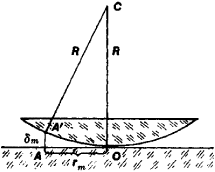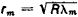# Newton's Rings

(redirected from Newtons rings)

## Newton's rings

[′nüt·ənz ′riŋz]
(optics)
A series of circular bright and dark bands which appear about the point of contact between a glass plate and a convex lens which is pressed against it and illuminated with monochromatic light.

## Newton’s Rings

equal-thickness interference fringes in the form of rings that are concentric with respect to the point of contact of two surfaces, such as two spheres or a plane and a sphere. Such rings were first described in 1675 by I. Newton.

The interference of light occurs in a narrow, usually air-filled, gap that separates the contiguous bodies; this gap acts as a thin film. Newton’s rings are observed both in transmitted and— more distinctly—in reflected light. With illumination by monochromatic light of the wavelengths λ, Newton’s rings are alternating dark and bright fringes. The bright fringes arise at points where the gap has a path difference equal to an integral number of wavelengths between the direct beam and the twice reflected beam (in transmitted light) or between beams reflected from both contiguous surfaces (in reflected light). Dark rings are formed where the number of wavelengths in the path difference of the rays is equal to a half-integer. The path difference is determined by the optical path length of a ray in the gap and by the change in phase of the light wave upon reflection.

For example, upon reflection from an air-glass interface, the phase changes by π, whereas upon reflection from a glass-air interface, the phase remains unchanged. Therefore, in the case of two glass surfaces, the m th dark Newton’s ring in reflected light corresponds to the path difference mλ, that is, a gap thickness of δm = mλ/2, where m is an integer.Figure 1.

Note to Figure 1: Derivation of relation among radii rm of Newton’s rings in reflected light, radius R of spherical lens, and wavelength λ of illuminating monochromatic light. O is the point of contact between the sphere and the plane and AA’ = δm is the thickness of the air gap in the region where the m th dark ring is formed. By applying the Pythagorean theorem to a right triangle whose short leg (equal to rm) represents the perpendicular dropped from A’ to CO, we obtain rm2 = R2 – (R – δm)2 ≈ 2Rδm. Hence the condition δm = λm/2 yieldsWhen the sphere and the plane are in contact (Figure 1), rm = (mλR)½. According to the Pythagorean theorem, for right triangles with legs rn and rm we have R2 = (R – λn/2)2 + rn2 and R2 = (R – λm/2)2 + rm2. Hence it follows that when very small terms, such as (mλ/2)2 and (nλ/2)2, are disregarded, the formula often used for Newton’s rings is R = (rn2rm2)/λ (nm). These relations make it possible either to determine λ from measured rm and rn with good accuracy or, if λ is known, to measure the radii of lens surfaces.

Newton’s rings are also used to monitor the accuracy of the shape of spherical and plane surfaces. With illumination by polychromatic light, such as white light, Newton’s rings become colored, and the sequence of colors in the rings differs significantly from the sequence in an ordinary rainbow because of the superpositioning of systems of rings corresponding to different m. Newton’s rings are observed most distinctly when spherical surfaces with small radii of curvature are used, since the thickness of the gap is small at a large distance from the point of contact.

### REFERENCES

Shishlovskii, A. A. Prikladnaia fizicheskaia optika. Moscow, 1961.
Ditchburn, R. Fizicheskaia optika. Moscow, 1965. (Translated from English.)

A. P. GAGARIN

Mentioned in ?
References in periodicals archive ?
Newtons Ring Apparatus to determine wavelength of sodium light,Set up to determine the wavelength of sodium light by Fresnel's biprism,Setup to determine wavelength of sodium light using diffraction grating & spectrometer,Set up to measure small thickness of a piece of paper using Newtons Rings technique,Setup to find e/m of an electron by J.
Tenders are invited for Procurement of Newtons rings apparatus,Travelling microscope,Fresnel Bi-prism Assembly Complete Setup,Spectrometer Kit,Polarimeter,HE - NE Laser Kit,Optical Fiber Trainer kit,e/m BY THOMPSON METHOD,Plank s constant trainer

Site: Follow: Share:
Open / Close